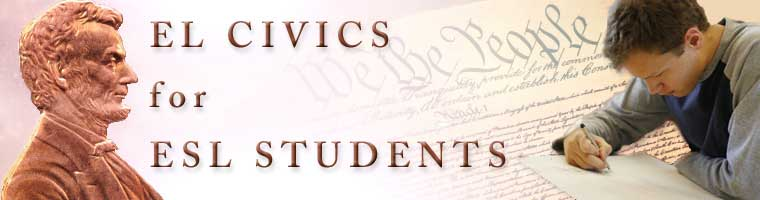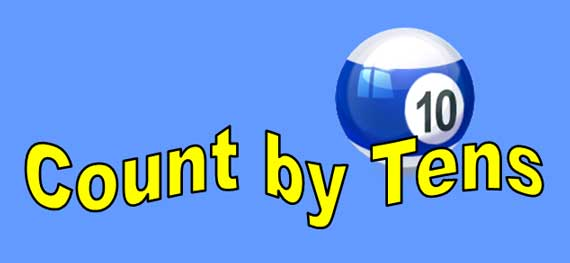Count by Tens Lesson

Counting by tens is easy: ten, twenty, thirty, forty, fifty, sixty, seventy, eighty, ninety, and one-hundred. All these numbers end with a zero. The first digits in the series increase by one. Counting by tens is useful when you count money that includes ten dollar bills or dimes. Cashiers and bank tellers need to count by tens quickly. (Beginning Level Lesson)

Ten

All numbers can be written with letters or with numerals.

ten = 10

0 + 10 = 10

Zero plus ten equal ten.

Twenty

twenty = 20

10 + 10 = 20

Ten plus ten equal twenty.